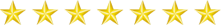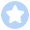CAN 总线（Controller Area Network）是控制器局域网的简称，是 20 世纪 80 年代初德国 BOSCH 公司为解决现代汽车中众多的控制与测试仪器之间的数据交换而开发的一种串行数据通信协议。目前，CAN 总线已经被列入 ISO 国际标准，称为 ISO11898。CAN 总线已经成为工业数据通信的主流技术之一。

CAN 总线作为数字式串行通信技术，与其他同类技术相比，在可靠性、实时性和灵活性方面具有独特的技术优势，主要特点如下：

•  CAN 总线是一种多主总线，总线上任意节点可在任意时刻主动地向网络上其他节点发送信息而不分主次，因此可在各节点之间实现自由通信。

•  CAN 总线采用非破坏性总线仲裁技术。但多个节点同时向总线发送信息时，优先级低的节点会主动退出发送，而最高优先级的节点可以不受影响地继续传输数据，从而大大节省总线冲突的仲裁时间。即使在网络负载很重的情况下也不会发生网络瘫痪情况。

• CAN 总线的通信介质可以是双绞线、同轴电缆或光导纤维，选择灵活。

• CAN 总线的通信速率可达 1Mbit/s（此时通信距离最长为 40 米），通信距离最远可达 10km（速率在 5kbit/s 以下）。

•  CAN 总线上的节点信息分成不同的优先级，可以满足不同级别的实时要求，高优先级的数据可以在 134μs 内得到传输。

• CAN 总线通过报文滤波即可实现点对点、一点对多点及全局广播等几种方式传送数据，无需专门的调度。

•  CAN 总线的数据采用短帧结构，传输时间短，受干扰概率低，具有极好的检错效果。

• CAN 总线采用 CRC 检验并可提供相应的错误处理功能，保证了数据通信的可靠性。

• CAN 总线上的器件可被置于无任何内部活动的睡眠方式，相当于未连接到总线上，可以有效降低系统功耗。

CAN 总线上的节点在错误严重的情况下具有自动关闭输出的功能，以使总线上其他节点的操作不受影响。CAN 总线卓越的特性、极高的可靠性和独特的设计，特别适合工业过程中监控设备的互连，因此，越来越受到工业界的重视，并被公认为是最有前途的现场总线之一。另外，CAN 总线协议已被国际标准化组织认可，技术比较成熟，控制的芯片已经商品化，性价比高，特别适用于分布式测控系统之间的数通讯。

CAN 总线插卡可以任意插在 PC AT XT 兼容机上，方便地构成分布式监控系统。因此，用 FPGA 实现 CAN 总线通信控制器具有非常重要的应用价值。本篇将通过一个实例讲解利用 FPGA 实现 CAN 总线通信控制器的实现方法。CAN 总线通信控制器的仿真程序，需要模拟数据的发送和接收。

``//连接 can_top 模块``  can_top i_can_top(``                    .cs_can_i(cs_can),``                    .clk_i(clk),``                    .rx_i(rx_and_tx),``                    .tx_o(tx),``                    .irq_on(irq),``                    .clkout_o(clkout)``                   );``​``//产生 24 MHz 时钟``  initial``  begin``    clk=0;``    forever #21 clk = ~clk;``  end``​``//初始化``  initial``  begin``    start_tb = 0;``    cs_can = 0;``    rx = 1;``    extended_mode = 0;``    tx_bypassed = 0;``    rst_i = 1'b0;``    ale_i = 1'b0;``    rd_i = 1'b0;``    wr_i = 1'b0;``    port_0_o = 8'h0;``    port_0_en = 0;``    port_free = 1;``    rst_i = 1;``    #200 rst_i = 0;``    #200 start_tb = 1;``  end``​``//产生延迟的 tx 信号(CAN 发送器延迟)``  always``  begin``    wait (tx);``    repeat (4*BRP) @ (posedge clk); // 4 time quants delay``    #1 delayed_tx = tx;``    wait (~tx);``    repeat (4*BRP) @ (posedge clk); // 4 time quants delay``    #1 delayed_tx = tx;``  end``  ``  assign rx_and_tx = rx & (delayed_tx | tx_bypassed); // When this signal is on, tx is not``  looped back to the rx.``  ``//主程序``  initial``  begin``    wait(start_tb);``​``//设置总线时序寄存器``    write_register(8'd6, {`CAN_TIMING0_SJW, `CAN_TIMING0_BRP});``    write_register(8'd7, {`CAN_TIMING1_SAM, `CAN_TIMING1_TSEG2, `CAN_TIMING1_TSEG1});``​``// 设置时钟分频寄存器``    extended_mode = 1'b0;``    write_register(8'd31, {extended_mode, 3'h0, 1'b0, 3'h0}); // Setting the normal mode (not``    extended)``​``//设置接收代码和接收寄存器``    write_register(8'd16, 8'ha6); // acceptance code 0``    write_register(8'd17, 8'hb0); // acceptance code 1``    write_register(8'd18, 8'h12); // acceptance code 2``    write_register(8'd19, 8'h30); // acceptance code 3``    write_register(8'd20, 8'h0); // acceptance mask 0``    write_register(8'd21, 8'h0); // acceptance mask 1``    write_register(8'd22, 8'h00); // acceptance mask 2``    write_register(8'd23, 8'h00); // acceptance mask 3``    write_register(8'd4, 8'he8); // acceptance code``    write_register(8'd5, 8'h0f); // acceptance mask``    #10;``    repeat (1000) @ (posedge clk);``  ``//开关复位模式``    write_register(8'd0, {7'h0, ~(`CAN_MODE_RESET)});``    repeat (BRP) @ (posedge clk);``  ``// 在复位后设置总线空闲``    repeat (11) send_bit(1);``    test_full_fifo; // test currently switched on``    send_frame; // test currently switched off``    bus_off_test; // test currently switched off``    forced_bus_off; // test currently switched off``    send_frame_basic; // test currently switched off``    send_frame_extended; // test currently switched off``    self_reception_request; // test currently switched off``    manual_frame_basic; // test currently switched off``    manual_frame_ext; // test currently switched off``    \$display("CAN Testbench finished !");``  \$stop;``  end``

``//强制关闭总线任务``task forced_bus_off; // Forcing bus-off by writinf to tx_err_cnt register``begin``//切换到复位模式``write_register(8'd0, {7'h0, `CAN_MODE_RESET});``// 设置时钟分频寄存器``write_register(8'd31, {1'b1, 7'h0}); // Setting the extended mode (not normal)``// 写数据到寄存器中``write_register(8'd15, 255);``// 切换复位模式``write_register(8'd0, {7'h0, ~(`CAN_MODE_RESET)});``#2500000;``// 切换复位模式``write_register(8'd0, {7'h0, `CAN_MODE_RESET});``// 写数据到寄存器中``write_register(8'd15, 245);``//关闭复位模式``write_register(8'd0, {7'h0, ~(`CAN_MODE_RESET)});``#1000000;``end``endtask // forced_bus_off``

``//发送一个基本格式的帧``task manual_frame_basic;``begin``// 切换到复位模式``  write_register(8'd0, {7'h0, (`CAN_MODE_RESET)});``//设置寄存器``  write_register(8'd4, 8'h28); // acceptance code``  write_register(8'd5, 8'hff); // acceptance mask``  repeat (100) @ (posedge clk);``// 切换复位模式``  write_register(8'd0, {7'h0, ~(`CAN_MODE_RESET)});``// 模块复位后设置总线空闲``  repeat (11) send_bit(1);``  write_register(8'd10, 8'h55); // Writing ID[10:3] = 0x55``  write_register(8'd11, 8'h57); // Writing ID[2:0] = 0x2, rtr = 1, length = 7``  write_register(8'd12, 8'h00); // data byte 1``  write_register(8'd13, 8'h00); // data byte 2``  write_register(8'd14, 8'h00); // data byte 3``  write_register(8'd15, 8'h00); // data byte 4``  write_register(8'd16, 8'h00); // data byte 5``  write_register(8'd17, 8'h00); // data byte 6``  write_register(8'd18, 8'h00); // data byte 7``  write_register(8'd19, 8'h00); // data byte 8``  tx_bypassed = 1; // When this signal is on, tx is not looped back to the rx.``  ``  fork``    begin``    self_reception_request_command;``    end``    ``    begin``      #2200;``      repeat (1)``      //开始发送数据``      begin``        send_bit(0); // 帧起始``        send_bit(0); // ID``        send_bit(1); // ID``        send_bit(0); // ID``        send_bit(1); // ID``        send_bit(0); // ID``        send_bit(1); // ID``        send_bit(0); // ID``        send_bit(1); // ID``        send_bit(0); // ID``        send_bit(1); // ID``        send_bit(0); // ID``        send_bit(1); // RTR``        send_bit(0); // IDE``        send_bit(0); // r0``        send_bit(0); // DLC``        send_bit(1); // DLC``        send_bit(1); // DLC``        send_bit(1); // DLC``        send_bit(1); // CRC``        send_bit(1); // CRC``        send_bit(0); // CRC stuff``        send_bit(0); // CRC 6``        send_bit(0); // CRC``        send_bit(0); // CRC``        send_bit(0); // CRC``        send_bit(1); // CRC stuff``        send_bit(0); // CRC 0``        send_bit(0); // CRC``        send_bit(1); // CRC``        send_bit(0); // CRC``        send_bit(1); // CRC 5``        send_bit(1); // CRC``        send_bit(0); // CRC``        send_bit(1); // CRC``        send_bit(1); // CRC b``        send_bit(1); // CRC DELIM``        send_bit(0); // ACK``        send_bit(1); // ACK DELIM``        send_bit(1); // EOF``        send_bit(1); // EOF``        send_bit(1); // EOF``        send_bit(1); // EOF``        send_bit(1); // EOF``        send_bit(1); // EOF``        send_bit(1); // EOF``        send_bit(1); // INTER``        send_bit(1); // INTER``        send_bit(1); // INTER``        end // repeat``      end``      ``    join``    //从接收缓冲中读取数据``    read_receive_buffer;``    release_rx_buffer_command;``    read_receive_buffer;``    release_rx_buffer_command;``    read_receive_buffer;``    #4000000;``    ``  end``endtask // manual_frame_basic``ENDFPGA工程师就业班，9月份开课！

### linux中svn-base的包怎么安装,安装软件包的三种方法 ，rpm包介绍 ， rpm工具用法， yum工具用法， yum搭建本地仓库..._SIY.Z的博客-程序员宅基地

linux安装和卸载软件安装三种方法rpm工具yum工具源码包rpm工具使用首先看一下什么事rpm包，可以挂载光驱看看里面有很多[root@localhost ~]# mount /dev/cdrom /mnt/mount: /dev/sr0 写保护，将以只读方式挂载[root@localhost ~]# df -h文件系统 容量 已用 可用 已用% 挂载点/dev/sda3 ...

### PDF转Word方法大盘点：看了这一篇，就不用再找转换技巧了-程序员宅基地

PDF文档成为正式文件之后，传输之间都是使用PDF文件。但是有部分的人仍不习惯编辑PDF文档，而适应Word编辑。这时转换就成了桥梁，将PDF文档转换成Word，即可以使用Word编辑文档，又可以接收PDF文档。今天小编就盘点了四种转换方法，希望能帮助到有需要的人。一、在线转换在线转换是最简单粗暴的方式，又不需要下载软件，就可以将PDF文件转换成Word。（1）在浏

### Swift 4.0 正式发布，更快更兼容更好用-程序员宅基地

Swift4现已正式发布！Swift4在Swift3的基础上，提供了更强大的稳健性和稳定性，为Swift3提供源码兼容性，对标准库进行改进，并添加了归档和序列化等功能。你可以通过观看WWDC2017:What’sNewinSwift或完整发行说明快速了解Swift4功能概述。一、语言更新StringSwift4包含一个更快、更易使用的String实...

### 【工具】m3u8转为mp4_m3u8转mp4 在线-程序员宅基地

author：咔咔wechat：fangkangfk直接下载，打开把你的m3u8地址存放进去就可以了，一分钟解决你的问题下载地址：https://www.yuque.com/u30882/rx39g7/zah4ef_m3u8转mp4 在线